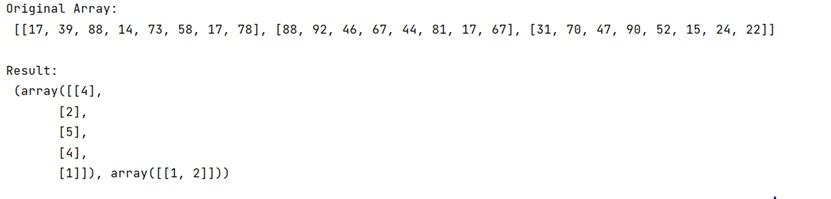# Index a 2D NumPy array with 2 lists of indices

Learn, how to index a 2D NumPy array with 2 lists of indices in Python?
Submitted by Pranit Sharma, on March 01, 2023

NumPy is an abbreviated form of Numerical Python. It is used for different types of scientific operations in python. Numpy is a vast library in python which is used for almost every kind of scientific or mathematical operation. It is itself an array which is a collection of various methods and functions for processing the arrays.

## Indexing a 2D NumPy array with 2 lists of indices

Suppose that we are given a 2D numpy array and we have 2 indexers one with indices for the rows, and one with indices for the column, we need to index this 2-dimensional numpy array with these 2 indexers.

For this purpose, we will use the numpy.ix_() with indexing arrays. This function is used to construct an open mesh from multiple sequences. This function takes N 1-D sequences and returns N outputs with N dimensions each, such that the shape is 1 in all but one dimension and the dimension with the non-unit shape value cycles through all N dimensions.

Let us understand with the help of an example,

## Python code to index a 2D NumPy array with 2 lists of indices

```# Import numpy
import numpy as np

# Creating a numpy array
arr =  [[17, 39, 88, 14, 73, 58, 17, 78],[88, 92, 46, 67, 44, 81, 17, 67],[31, 70, 47, 90, 52, 15, 24, 22]]

# Display original array
print("Original Array:\n",arr,"\n")

# Defining indexers
row = [4, 2, 5, 4, 1]

col = [1, 2]

# Indexing 2D array
res = np.ix_(row,col)

# Display result
print("Result:\n",res,"\n")
```

Output:Languages: » C » C++ » C++ STL » Java » Data Structure » C#.Net » Android » Kotlin » SQL
Web Technologies: » PHP » Python » JavaScript » CSS » Ajax » Node.js » Web programming/HTML
Solved programs: » C » C++ » DS » Java » C#
Aptitude que. & ans.: » C » C++ » Java » DBMS
Interview que. & ans.: » C » Embedded C » Java » SEO » HR
CS Subjects: » CS Basics » O.S. » Networks » DBMS » Embedded Systems » Cloud Computing
» Machine learning » CS Organizations » Linux » DOS
More: » Articles » Puzzles » News/Updates